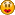# October Handicapping

Im a bit math challenged, but heres my results-

3 days
12 wins=3000
7 place=700
7 shows=350
4 exacta=2000

Total=6050

Dawn
Battle Hill Farm

Days Handicapped: 8
Win: 36 x \$250 = \$9000
Place: 20 x \$100 = \$2000
Show: 20 x \$50 = \$1000
Quinella: 10 x \$500 = \$5000
Trifecta: 5 x \$1000 = \$5000
Total: \$22,000

Heh, I was lazy this month.Name: Nan
Stable: Lanson Farms

Total days handicapped: 1
Total wins: 8 x \$250 = \$2000
Total places: 1 x \$100 = \$100
Total shows: 2 x \$50 = \$100
Total money won: \$2200

Name:Cyanne Black
Stable: Black Diamond Estates

Total Days Handicapped:7
Wins: 30 x \$250 = \$7500
Places: 16 x \$100 = \$1600
Shows: 20 x \$50 = \$1000
Trifecta: 8 x \$1000 = \$8000
Quinella: 6 x \$500 = \$3000
Total: \$19,600

Name: Sarah
Stable: Blue Wolf Farm

Total Days Handicapped: 8
Win: 36 x \$250 = \$9,000
Place: 33 x \$100 = \$3,300
Show: 29 x \$50 = \$1,450
Quinella: 6 x \$500 = \$3,000
Trifecta: 3 x \$1000 = \$3,000
Total: \$19,750

Did better than last month.Name: LeAnn
Stable: Freedom Acres

Total Days Handicapped: 2
Win: 11 x \$250 = \$2,750
Place: 8 x \$100 = \$800
Show: 6 x \$50 = \$300
Quinella: 2 x \$500 = \$1,000
Trifecta: 2 x \$1,000 = \$2,000
Total: \$6,850

Krystal
Menelya

Total Days Handicapped: 1
Win: 4 x \$250 = \$1000
Place: 5 x \$100 = \$500
Show: 2 x \$50 = \$100
Quinella: 2 x \$500 = \$1000
Trifecta: 1  x \$1000 = \$1000
Total: \$3600

Bame
Wither Hawk Stables

Days Handicapped: 2
Win: 5 x \$250 = \$1,250
Place: 9 x \$100 = \$900
Show: 6 x \$50 = \$300
Quinella: 3 x \$500 = \$1,500
Trifecta: 2 x \$1000 = \$2,000
Total: \$5950

The above money has been handed out.  For future reference, if it’s been more than 3 weeks since the month ended and I haven’t handed out \$, feel free to shoot me a PM and remind me.I tend to ignore this forum because I never do handicapping.